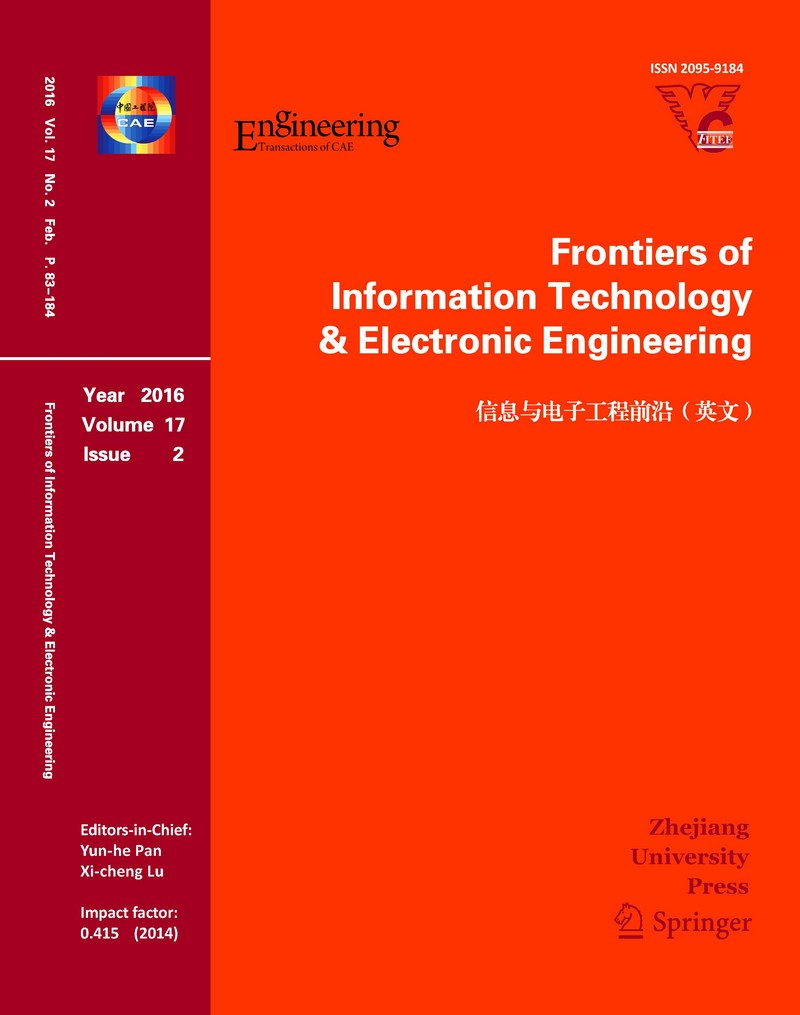,     et al.

,     et al.

,     et al.

,     et al.

,     et al.

,     et al.

,     et al.

,     et al.

,     et al.

,     et al.

,     et al.

,     et al.

,     et al.

,     et al.

,     et al.

,     et al.

,     et al.

,     et al.

,     et al.

,     et al.

,     et al.

,     et al.

#### Effective remediation of organic-metal co-contaminated soil by enhanced electrokinetic-bioremediation process

,     et al.#### Frontiers of Information Technology & Electronic Engineering

Volume 22,  Issue 3

,     et al.

,     et al.

,     et al.

,     et al.

,     et al.

,     et al.

,     et al.

,     et al.

,     et al.

,     et al.

,     et al.

,     et al.

,     et al.

,     et al.

,     et al.

,     et al.